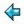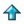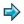### kate.lit.m

\documentclass[10pt]{article}
\title{{\tt kate.lit.m} --- KaTify text}
\author{J. Cupitt}
\date{July 21st, 1989}
% turn off para indents
\setlength{\parindent}{0in}
% put some space between paras
\setlength{\parskip}{0.05in}
\begin{document}
\maketitle

%An example of a Miranda literate script that is also a LaTeX source
%file.  Note that the lines of formal program text are surrounded by
%LaTeX verbatim directives.  Contributed by John Cupitt, of the
%University of Kent.

There is a group on USENET called \verb"rec.music.gaffa", dedicated to the
singer Kate Bush. A running joke in this group is to pretend fanatical
devotion to Kate And Her Works --- this reaches an extreme form in some
posters, who capitalise all the Ks and Ts in their articles in reference both
to KaTe herself and to the Knights Templar. This Miranda\footnote{Miranda is a
trademark of Research Software Ltd.} script can be used as a {\sc
Unix}\footnote{UNIX is a trademark of AT\&T in the USA and other
countries.} filter to prepare your articles for posting to \verb"gaffa".
The main function is called \verb"kate" and is at the end.

Do some simple maps on text. We do:

\begin{center}
\begin{tabular}{rcl}
c,C,k & $\rightarrow$ & K \\
t & $\rightarrow$ & T \\
qu,Qu & $\rightarrow$ & Kw \\
ck & $\rightarrow$ & K \\
ch,Ch & $\rightarrow$ & Khe
\end{tabular}
\end{center}

We also look for Kommon words that can be easily swapped for something with
more ks and ts.

The dictionary we use to look for common words. This is very small at the
moment! I should perhaps find a thesaurus and fix this up properly.

\begin{verbatim}

> kateMap
>      = [(["interpose", "insert"],
>                "interject"),
>         (["frequent", "general", "usual", "normal"],
>                "common"),
>         (["program", "file"],
>                "script"),
>         (["name"],
>                "appelation"),
>         (["however"],
>                "though"),
>         (["serve"],
>                "officiate"),
>         (["intersperse"],
>                "punctuate")
>        ]

\end{verbatim}

First map. Very easy!

\begin{verbatim}

> swapCase :: [char] -> [char]
> swapCase ('c':'k':x) = 'K':swapCase x
> swapCase ('c':'h':x) = 'K':'h':'e':swapCase x
> swapCase ('C':'h':x) = 'K':'h':'e':swapCase x
> swapCase ('c':x) = 'K':swapCase x
> swapCase ('C':x) = 'K':swapCase x
> swapCase ('k':x) = 'K':swapCase x
> swapCase ('t':x) = 'T':swapCase x
> swapCase ('q':'u':x) = 'K':'w':swapCase x
> swapCase ('Q':'u':x) = 'K':'w':swapCase x
> swapCase (a:x) = a: swapCase x
> swapCase [] = []

\end{verbatim}

Second map. We loop down the input again, chopping out words. Each one gets
put through tryMap.

\begin{verbatim}

> swapWords :: [char] -> [char]
> swapWords [] = []
> swapWords inp
>      = punk ++ tryMap word ++ swapWords tail
>        where
>        punk = takewhile ((~) . letter) inp
>        start = dropwhile ((~) . letter) inp
>        word = takewhile letter start
>        tail = dropwhile letter start

\end{verbatim}

Try to map a word through the KaTe thesaurus we defined earlier. We try to be
clever about what we swap. For example, we want \verb"insert" to be mapped to
\verb"interject", and \verb"inserting" to be mapped to \verb"interjecting".
This isn't always the most sensible way to do it \ldots

\begin{verbatim}

> tryMap :: [char] -> [char]
> tryMap word
>      = word, if maps = []
>      = hd maps, otherwise
>        where
>        maps = [ to ++ drop (#x) word |
>                        (from, to) <- kateMap; x <- from;
>                        x \$isprefix word ]

\end{verbatim}

Test for first argument a prefix of the second argument.

\begin{verbatim}

> isprefix :: [*] -> [*] -> bool
> isprefix a b = take (#a) b = a

\end{verbatim}

And our entry point. We just pipe stuff first through swapWords, then through
swapCase.

\begin{verbatim}

> kate :: [char] -> [char]
> kate = swapCase . swapWords

\end{verbatim}
\end{document}